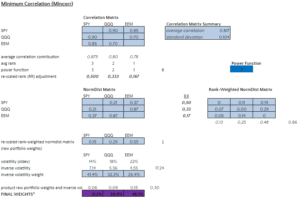# Minimum Correlation Portfolio

The minimum correlation portfolio is a heuristic portfolio allocation algorithm that aims to construct a portfolio that is well-diversified. The portfolio approach was developed by David Varadi, Michael Kapler, an Henry Bee in 2012.

On this page, we discuss the main methodology behind the minimum correlation portfolio. Because the approach is based on an algorithm, it is not easy to apply the approach using Excel, at least not for a large set of securities. Instead, implementations of the approach in R and Python are available.

An Excel implementation of the algorithm for three securities is available on the website of the authors of the paper.

## Minimum correlation intuition

The objective of the minimum correlation algorithm is to choose the portfolio weights such that the assets are weighted proportionally to their average correlation with the other assets in the portfolio. That way, we obtain a portfolio where assets that have lower correlation to all the other assets in the portfolio get a higher weight.

## Minimum correlation algorithm

Now that we have discussed the intuition, let’s turn to the algorithm itself

• Step 1: calculate the correlation matrix
• Step 2: put the correlations on a relative scale (such that positive and negative correlations can be compared
• step 3: rank-weight securities based on their average correlation (positions with a lower average correlation get a higher weight)
• step 4: normalise the volatility of each security such that the have equivalent risk in the portfolio

## Minimum correlation example

The following figure illustrates the application of the minimum portfolio approach using spreadsheet software and three securities. The spreadsheet itself is available on the authors’ website here.## Summary

We discussed the minimum correlation algorithm. This approach is similar to risk parity, which is also a portfolio construction approach that uses only the covariance matrix to construct a portfolio. Another related approach is the maximum diversification approach.